• 一种基于机器学习的属性缺失值模糊填补方法.pdf
• 提出了一种基于灰色系统理论的缺失值填补方法，该方法采用了基于实例学习的非参拟合和灰色理论技术，对缺失数据进行重复填补，直至填补结果收敛或者满足用户的需要。实验结果表明，该方法在填补效果与效率方面都比...
• 介绍对于处理现实中的数据时，我们...其他类似的缺失值填补方法还有MICE，在这里不做介绍。方法我们假设我们的数据是X=(X1,X2,...,Xp)X=(X_1,X_2,...,X_p)的n*p的一个矩阵。如果对于一个任意的变量XsX_s，我们想填充
#介绍 对于处理现实中的数据时，我们常常会遇到缺失值，这里我们将介绍一种缺失值的填补方法missForest，这是利用随机森林来填补缺失值的非参数方法，他可以适用于任何类型的数据(连续、离散)。其他类似的缺失值填补方法还有MICE，在这里不做介绍。 #方法 我们假设我们的数据是

X

=

(

X

1

,

X

2

,

.

.

.

,

X

p

)

X=(X_1,X_2,...,X_p)

的n*p的一个矩阵。如果对于一个任意的变量

X

s

X_s

，我们想填充他的缺失值，我们可以将数据分成4部分： 1.用

y

o

b

s

(

s

)

y^{(s)}_{obs}

表示

X

s

X_s

的观测值。 2.用

y

m

i

s

(

s

)

y^{(s)}_{mis}

表示

X

s

X_s

的缺失值。 3.用

x

o

b

s

(

s

)

x^{(s)}_{obs}

表示

X

s

X_s

以外的观测值。 4.用

x

m

i

s

(

s

)

x^{(s)}_{mis}

表示

X

s

X_s

的缺失值以外的其余观测值。
我画了一个图，可以帮助大家更好的理解这4个部分的组成：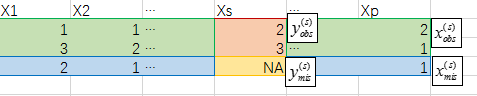红色部分就是

y

o

b

s

(

s

)

y^{(s)}_{obs}

，黄色部分是

y

m

i

s

(

s

)

y^{(s)}_{mis}

，绿色部分是

x

o

b

s

(

s

)

x^{(s)}_{obs}

，蓝色部分是

x

m

i

s

(

s

)

x^{(s)}_{mis}

。 接来下我们只需要使用随机森林，训练出y~x的模型，然后将缺失值预测出来就可以了，但是不只是Xs存在缺失值，其他变量也是有可能存在缺失值的，这时候我们可以通过迭代的方式来求解。 我们先对缺失值做一个初始的猜测，比如用均值/中位数填充，然后按照变量的缺失率，从小到大排序，先对缺失率小的变量使用随机森林回归从而填补该变量的缺失值，然后一直迭代，直到最新的一次填补结果与上一次的填补结果不再变化（变化很小）时停止。
具体伪代码在这里：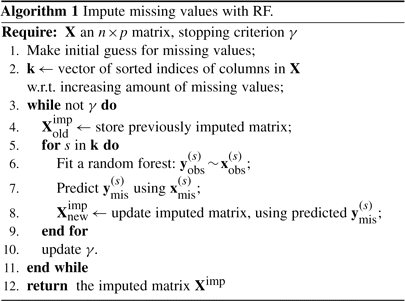这里的收敛指标是迭代中缺失值变化的大小，对于连续型变量，我们有：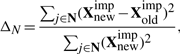对于离散型变量：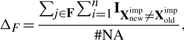其中#NA是在离散变量中的总的缺失值数量。
#R语言实现应用 我们可以使用R包：missForest 来应用这一方法： 以下是一个使用例子：
> library(missForest)
> set.seed(81)
> iris.mis <- prodNA(iris, noNA = 0.2) #产生20%缺失值
> summary(iris.mis)
Sepal.Length    Sepal.Width     Petal.Length    Petal.Width          Species
Min.   :4.300   Min.   :2.000   Min.   :1.100   Min.   :0.100   setosa    :40
1st Qu.:5.200   1st Qu.:2.800   1st Qu.:1.600   1st Qu.:0.300   versicolor:38
Median :5.800   Median :3.000   Median :4.450   Median :1.300   virginica :44
Mean   :5.878   Mean   :3.062   Mean   :3.905   Mean   :1.222   NAs      :28
3rd Qu.:6.475   3rd Qu.:3.300   3rd Qu.:5.100   3rd Qu.:1.900
Max.   :7.900   Max.   :4.400   Max.   :6.900   Max.   :2.500
NAs   :28      NAs   :29      NAs   :32      NAs   :33

> iris.imp <- missForest(iris.mis, verbose = TRUE)
> iris.imp$ximp #修补后的数据 > head((iris.imp$ximp-iris)[,1:4])
Sepal.Length Sepal.Width Petal.Length Petal.Width
1   0.00000000     0.00000   0.00000000  0.00000000
2   0.00000000     0.30825   0.00000000  0.02720000
3   0.00000000     0.00000   0.00000000  0.00000000
4   0.00000000     0.22050  -0.03142857  0.00000000
5   0.00000000     0.00000   0.00000000  0.00000000
6   0.01766667     0.00000   0.00000000 -0.02533333

可以看到修补的数据与原数据想减，我们就可以清楚的看到这个效果是很不错的，如果我们仅仅使用均值这样的填充方法，就不能够这么准确了。
当然这方法虽然效果比较好，但是相比均值填充的方法来讲，效率就太低了，如果数据量比较大的话，这个方法会很慢，至于如何使用，就看各位自己的取舍了。 #参考文献： Stekhoven D J, Bühlmann P. MissForest–non-parametric missing value imputation for mixed-type data.[J]. Bioinformatics, 2012, 28(1):112-8.

作为分享主义者(sharism)，本人所有互联网发布的图文均遵从CC版权，转载请保留作者信息并注明作者a358463121专栏:http://blog.csdn.net/a358463121，如果涉及源代码请注明GitHub地址：https://github.com/358463121/。商业使用请联系作者。

展开全文缺失值 R语言 随机森林
• 如何寻找使用的数据集最佳的缺失值填补方法（分析思路的整理）： 第一步，导入需要用到的库； 第二步，导入完整的数据集并进行探索，以波士顿数据为例 例如：将特征数据集和标签数据集分出来 X_full, y_full...
寻找数据集最佳的缺失值的填补方法（分析思路的整理）：
第一步，导入需要用到的库；
第二步，导入完整的数据集并进行探索，以波士顿数据为例
例如：将特征数据集和标签数据集分出来

X_full, y_full = dataset.data, dataset.target
# 找出特征列的的行列的范围

n_samples
=
X_full
.
shape
[
0
]

n_features
=
X_full
.
shape
[
1
]

第三步，为完整数据集放入缺失值
首先设置一个缺失的比例，计算出缺失的数据的数量
然后从特征的行列索引范围内，随机取要选的数量

missing_features = rng.randint(0,n_features,n_missing_samples) # randint(下限，上限，n)  ,意思是请在下限和上限之间取出n个整数
missing_samples = rng.randint(0,n_samples,n_missing_samples)

最后，防止操作错误，先复制数据集，然后，将选出的索引值的位置用nan填充

X_missing = X_full.copy() y_missing = y_full.copy()
X_missing[missing_samples,missing_features] = np.nan

第四步，  使用0和均值填补缺失值
第一种：使用均值填补缺失值

#使用均值进行填补 from sklearn.impute import SimpleImputer imp_mean = SimpleImputer(missing_values=np.nan, strategy='mean') # 实例化 X_missing_mean = imp_mean.fit_transform(X_missing)               # 训练fit+导出predict 》》》特殊的接口fit_transform

第二种：使用0去填充

# 数据用0进行填充 imp_0 = SimpleImputer(missing_values=np.nan, strategy="constant",fill_value=0)  # constant 是指常数 X_missing_0 = imp_0.fit_transform(X_missing)

第五步，使用随机森林填补缺失值
首先，将原始的特征列复制一份数据集，找出数据集中，缺失值从小到大排列的特征们的顺序

X_missing_reg = X_missing.copy()
# 找出数据集中，缺失值从小到大排列的特征们的顺序,有了这些特征的索引 sortindex = np.argsort(X_missing_reg.isnull().sum(axis=0)).values # np.sort()会返回从小到大排序的值，会丢掉索引 # np.argsort()会返回从小到大排序的顺序所对应的索引，数组array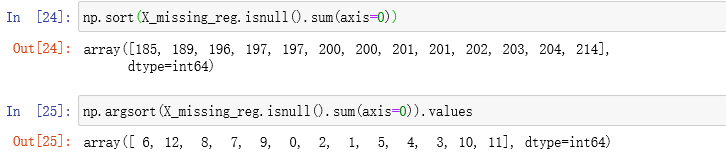然后，做循环（整个过程大致可以分为五步），从缺失值最大的开始。
参考代码：
for i in sortindex:
#构建我们的新特征矩阵（没有被选中去填充的特征+原始的标签）和新标签（被选中去填充的标签）
df = X_missing_reg   # 目的是为了在进行循环时，缺失的特征值用0的填充，不改变原有的数据集
fillc = df.iloc[:,i]
df = pd.concat([df.iloc[:,df.columns != i],pd.DataFrame(y_full)],axis=1)
#在新特征矩阵中，对含有缺失值的列，进行0的填补；先实例化，后接口，一步到位
df_0 =SimpleImputer(missing_values=np.nan,
strategy='constant',fill_value=0).fit_transform(df)
#找出我们的训练集和测试集
Ytrain = fillc[fillc.notnull()]
Ytest = fillc[fillc.isnull()]
Xtrain = df_0[Ytrain.index,:]
Xtest = df_0[Ytest.index,:]
#用随机森林回归来填补缺失值
rfc = RandomForestRegressor(n_estimators=100)
rfc = rfc.fit(Xtrain, Ytrain)
Ypredict = rfc.predict(Xtest)
#将填补好的特征返回到我们的原始的特征矩阵中，用切片和索引来做
X_missing_reg.loc[X_missing_reg.iloc[:,i].isnull(),i] = Ypredict
第六步， 对填补好的数据进行建模
对所有的数据进行建模，取得mse结果
#对所有数据进行建模，取得MSE结果
X = [X_full,X_missing_mean,X_missing_0,X_missing_reg]
mse = []
for x in X:
estimator = RandomForestRegressor(random_state=0, n_estimators=100) # shilihua
scores = cross_val_score(estimator,x,y_full,scoring='neg_mean_squared_error',
cv=5).mean()
mse.append(scores * -1)
第七步，用所画的结果画出条形图
通过在条形图上进行对比，观察四种情况之下的拟合状况。
展开全文• 在我们进行数据分析时，通常会遇到各种数据缺失...对某一列的缺失值，采用该列的平均值填充 df.fillna(method=a.mean(),inplace=True) 此处重点讲解一下fillna的参数，后面不做说明 fillna(value=None, method=...
在我们进行数据分析时，通常会遇到各种数据缺失的情况，针对这种情况我们该如何进行填补呢？
1、人工填补
该方法仅适用于小数据集，受个人因素影响。
2、平均值填补
对某一列的缺失值，采用该列的平均值填充
df.fillna(method=a.mean(),inplace=True)
此处重点讲解一下fillna的参数，后面不做说明
fillna(value=None, method=None, axis=None, inplace=False, limit=None, downcast=None, **kwargs)
#value：固定值，可以用固定数字、均值、中位数、众数等，此外还可以用字典，series等形式数据；
#axis: 填充方向，默认0和index，还可以填1和columns
#inplace:在原有数据上直接修改
#limit:填充个数，如1，每列只填充1个缺失值


3、临近值填补
对每一列的缺失值，采用临近位置的数据进行填充。
#使用每列缺失值前面的值进行填充(按相应列填充,按照相应index前后填充)
df.fillna(method='ffill',inplace=True)
#使用每列缺失值后面的值进行填充(按相应列填充,按照相应index前后填充)
df.fillna(method='backfill',inplace=True)
df.fillna(method='bfill',inplace=True)
#使用每列缺失值前面的值进行填充(按相邻列填充,按照相应columns前后填充)
df.fillna(method='ffill',inplace=True,axis=1)
#使用每列缺失值后面的值进行填充(按相邻列填充,按照相应columns前后填充)
df.fillna(method='backfill',inplace=True,axis=1)
df.fillna(method='bfill',inplace=True,axis='columns')
对每一列的缺失值，采用临近位置上下两个值的平均值来填充
#上下两个值的平均值进行填充
df=df.fillna(df.interpolate())
#在此详细介绍下interpolate函数的参数：'nearest', 'zero', 'slinear', 'quadratic', 'cubic', 'spline', 'barycentric', 'polynomial'
4、中位数填补
对每列的数值采用中位数进行填补
df.fillna(df.median(),inplace=True)
5、众数填补
对每列数值采用众数填补
df.fillna(df.mode(),inplace=True)
6、回归填补
对于有缺失值的特征值，将已知特征值代入模型来估计未知特征值，以此估计值来进行填充，该填充方法适用于缺失值为定量的数据类型。
from sklearn.experimental import enable_iterative_imputer
from sklearn.impute import IterativeImputer
#data为缺失的数据集
imp = IterativeImputer(max_iter=10, random_state=0)
imp.fit(data)

print(np.round(imp.transform(data)))
7、热平台填补
热平台插补又被称为热卡插补，是从每一缺失数据的估计分布抽取插补值来取代缺失值，使用回答单元中的抽样分布作为抽样分布是常见的方法。热卡插补包括随机抽样插补、分层热卡插补、最近距离热卡插补和序贯热卡插补。
随机抽样插补。缺失值为Missing Completely at Random，MCAR，即完全随机缺失的情况下，对某个确实字段进行简单随机抽样、限制对回答单元的使用次数、对回答单元进行排序并进行抽样；
分层热卡填补。首先按照某些辅助变量对y进行分层，然后对分层的数据进行热卡插补；
最近距离热卡插补。利用辅助变量，定义一个测量单元间距离的函数，在变量y的无回答单元临近的回答单元中，选择满则设定的距离条件的辅助变量中的单元所对应的变量y的回答单元作为插补值。由于使用较为复杂的距离函数，很难对插补后的均值和方差等估计量的性质进行考察；
序贯热卡填充。基于最近距离热卡插补来实现的，首先对数据分层，在每层中按照选定的某个辅助变量排序，并在其前后相邻的10个数据中，找到是的设定的某一个距离函数的值达到最小的单元，使用该单元对应的变量y的回答单元作为插补值。
8、冷平台填补
从以前的调查中或其他信息来源中获得，如历史数据。
9、极大似然估计
缺失类型为随机缺失的条件下，假设模型对于完整的样本是正确的，那么通过观测数据的边际分布可以对未知参数进行极大似然估计。这种方法也被称为忽略缺失值的极大似然估计，对于极大似然的参数估计实际中常采用的计算方法是期望值最大化。该方法适用于大样本。
10、k均值聚类法
该方法又被称为K最近距离邻法。实现方式为：采用k-means聚类将所有样本进行聚类划分，然后再通过划分的种类的均值对各自类中的缺失值进行填补。
11、随机森林填补
采用随机森林来对缺失值进行拟合。
from  sklearn import ensemble
from sklearn.preprocessing import LabelEncoder
def set_missing(df,estimate_list,miss_col):
"""df要处理的数据帧，estimate_list用来估计缺失值的字段列表,miss_col缺失字段名称;会直接在原来的数据帧上修改"""
col_list=estimate_list
col_list.append(miss_col)
process_df = df.loc[:,col_list]
class_le= LabelEncoder()
for i in col_list[:-1]:
process_df.loc[:,i]=class_le.fit_transform(process_df.loc[:,i].values)
# 分成已知该特征和未知该特征两部分
known=process_df[process_df[miss_col].notnull()].values
known[:, -1]=class_le.fit_transform(known[:, -1])
unknown = process_df[process_df[miss_col].isnull()].values
# X为特征属性值
X = known[:, :-1]
# y为结果标签值
y = known[:, -1]
# fit到RandomForestRegressor之中
rfr = ensemble.RandomForestRegressor(random_state=1, n_estimators=200,max_depth=4,n_jobs=-1)
rfr.fit(X,y)
# 用得到的模型进行未知特征值预测
predicted = rfr.predict(unknown[:, :-1]).round(0).astype(int)
predicted=class_le.inverse_transform(predicted)
#     print(predicted)
# 用得到的预测结果填补原缺失数据
df.loc[(df[miss_col].isnull()), miss_col] = predicted
return df

#还可以用决策树做拟合
from sklearn import tree
dt=tree.DecisionTreeRegressor()
dt.fit(X,y)
predicted = clf.predict(unknown[:, :3]).round(0)
predicted=class_le.inverse_transform(predicted)
print(predicted)
12、趋势得分法
13、马尔科夫链蒙德洛夫法
14、随机回归填补
15、增加虚拟变量
在原有数据集上增加一个字段。判断特征值是否有缺失值来定义一个新的二分类变量
展开全文• 我是做医学相关的预测模型，收集到的数据有很多缺失值，比如像白蛋白、血钠、血磷等等这些数据，看到有很多缺失值填补方法是，匀称分布的用的是均值，非匀称分布的是中位数。但是用这样填补的数据，做模型效果会不会...机器学习
• from sklearn.preprocessing import Imputer 填补缺失值：sklearn.preprocessing.Imputer(missing_values=’NaN’, strategy=’mean’, axis=0, ...missing_values：缺失值可以为整数或NaN(缺失值numpy.n...
from sklearn.preprocessing import Imputer

填补缺失值：sklearn.preprocessing.Imputer(missing_values=’NaN’, strategy=’mean’, axis=0, verbose=0, copy=True)
主要参数说明：
missing_values：缺失值，可以为整数或NaN(缺失值numpy.nan用字符串‘NaN’表示)，默认为NaN
strategy：替换策略，字符串，默认用均值‘mean’替换
①若为mean时，用特征列的均值替换
②若为median时，用特征列的中位数替换
③若为most_frequent时，用特征列的众数替换
axis：指定轴数，默认axis=0代表列，axis=1代表行
copy：设置为True代表不在原数据集上修改，设置为False时，就地修改，存在如下情况时，即使设置为False时，也不会就地修改

展开全文• pandas填补缺失值方法 在处理数据的过程中，经常会遇到原数据部分内容的缺失，为了保证我们最终数据统计结果的正确性，通常我们有两种处理方式，第一种就是删除掉这些部分缺失的数据；第二种就是填补这些缺失的...pandas 数据分析 python
• 对于两端缺失数据使用前后填充，对于中间数据使用线性插值（可替换） import numpy as np import pandas as pd from scipy.interpolate import interp1d df = pd.read_excel('data.xlsx') df['城市'].fillna...
• 填补缺失值使用0或均值填补缺失值使用随机森林填补缺失值 使用0或均值填补缺失值 #使用均值进行填补 from sklearn.impute import SimpleImputer #引入sklearn中用来填充的类 imp_mean = SimpleImputer(missing_...机器学习
• ## 数据缺失值填补

千次阅读 2018-10-15 17:20:34
缺失值填充方法包括简单填充、属性填充和实例填充三种类型，本文主要研究属性填充方式。 填充依赖原始数据的单一或少量属性维度，利用属性间的关系或属性潜在的规则对缺失值进行填充。 常用的属性填充方法有:基于...数据处理
• 行业分类-物理装置-基于改进马尔可夫模型和改进K最近邻的缺失值填补方法.zip
• 行业分类-物理装置-基于交替学习的不完整数据精细建模及缺失值填补方法.zip
• 行业分类-物理装置-一种不均衡不完整数据集的建模及缺失值填补方法.zip
• SAS中没有专门的处理缺失值的过程步，但是在标准化变量的过程步中可以实现缺失值的填补。其基本过程如下： ...其中proc stdize 是调用标准化过程步，data=表示需要进行缺失值填补的数据库，reponly的意思是只取代缺
• ## R语言填补缺失值

千次阅读 2020-12-25 21:03:20
下面将介绍如何进行缺失值填补的各种方法 用某特定值替换缺失值 下面这段代码表示使用0填补缺失值，x是需要填补的数据框的某行或某列，如果是其他值，将0改成需要的值即可。 FillNA <- function(x){ x[is.na(x )...r语言
• 采用目标信号相位差和...该方法不仅具有较精确的填补能力,而且不受缺失值分布情况的限制,保证了测量值的完整性和准确性,实现了相位差变化率定位法的快速高精度定位。仿真结果表明,该方法在5s内的相对定位误差为2.14%。
• 填补缺失值方法其实有很多，利用pandas自带的fillnan,replace方法，使用sklearn.impute的SimpleImputer等都是可以填补的，在这里主要是介绍使用随机森林来进行填补缺失值。 利用随机森林填补缺失值 利用随机森林...
• Pandas填充缺失值两种方法：bfill/ffill对比...